## 30 January 2009

### Honeycomb panels 03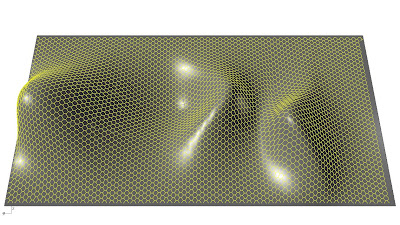Honeycomb tesselation rhinoscript optimized:
-contouring curves created by rhinoscript
-equal distance of contouring curves is variable
-selection of curves more fast, not one by one
-number of division points is variable
-more fast honeycomb construction

Option Explicit
' Author: Milutin Cerovic 2009
' www.4ofseven.com
Call Main()
Sub Main()
'---------------------
'declare variables
'---------------------
Dim arrPoints
Dim i,j
Dim strObject, arrStartPoint, arrEndPoint, arrContours, divnumber
Dim arrIsoCurves
Const rhObjectCurve = 4
'---------------------
'select surface or Polysurface
'---------------------
strObject = Rhino.GetObject("Select object", rhObjectSurface + rhObjectPolysurface)
'define plane of section
'---------------------
arrStartPoint = Rhino.GetPoint("select contour base plane startpoint")
arrEndPoint = Rhino.GetPoint("select contour plane endpoint", arrStartPoint)
'---------------------
'define distance of sections
'---------------------
divnumber= rhino.GetReal("enter equal distance of sections contour")
arrContours = Rhino.SurfaceContourPoints(strObject, arrStartPoint, arrEndPoint,divnumber)
'---------------------
'draw curve through section arraypoints
'---------------------
If IsArray(arrContours) Then
For Each arrPoints In arrContours
Next

End If
'---------------------
'select isocurves
'---------------------
arrIsoCurves=Rhino.GetObjects ("Select Section curves, from left to right or viceversa",rhObjectcurve)
ReDim arrPoints(UBound(arrIsoCurves))
'---------------------
'divide curve into equal distances and array points
'-------------------------------
DivNumber = Rhino.GetReal ("enter divide number curves")
For i=0 To UBound(arrIsoCurves)
arrPoints(i)=Rhino.DivideCurve(arrIsoCurves(i),DivNumber,True)
Next
'---------------------
'draw lines with honeycomb pattern
'-------------------------------
'>>>>>>>>>>>>>ACTIVE NEXT LINE FOR FAST CALCULATION<<<<<<<<<<<<<<<<<<

' Call rhino.EnableRedraw (False)
For i=0 To (UBound(arrIsoCurves)-1)Step 4
For j=0 To (DivNumber-2)
If j Mod 2 <> 0 Then

End If
Next
Next
'>>>>>>>>>>>>>ACTIVE NEXT LINE FOR FAST CALCULATION<<<<<<<<<<<<<<<<<<

'Call rhino.EnableRedraw (True)
End Sub

## 29 January 2009

### Honeycomb panels 02After last post you can found here, where i used rhino paneling tool in order to reconstruct a surface with honeycomb tesselation, now i wrote a very simple lines construction point by point in a rhinoscript found on this blog: 4ofseven, Design Studio at University of Belgrade Graduate Programme.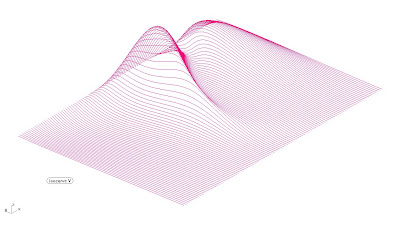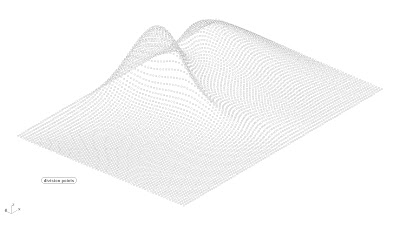Option Explicit
' Author: Milutin Cerovic 2009
' www.4ofseven.com

Call Main()
Sub Main()
'declare variables
'-------------------------------

Dim arrIsoCurves
Dim arrPoints
Dim DivNumber
Dim i,j

'select isocurves
'-------------------------------
arrIsoCurves=Rhino.GetObjects ("Select Isocurves, in order one by one")
ReDim arrPoints(UBound(arrIsoCurves))
'divide curve into equal distances and array points

'-------------------------------
DivNumber = Rhino.GetReal ("enter divide number
")
For i=0 To UBound(arrIsoCurves)
arrPoints(i)=Rhino.DivideCurve(arrIsoCurves(i),DivNumber,True)

Next
'draw lines with honeycomb pattern
'-------------------------------

For i=0 To (UBound(arrIsoCurves)-1)Step 4
For j=0 To (DivNumber-2)
If j Mod 2 <> 0 Then

End If
Next
Next
End Sub### Diagrid bookshelf 01Working on rhino grasshoper file using boolean difference in order to create shelves that follow the diagrid tesselation on a surface. In another test i worked on aperture of diagrid cells through a point attractor that control the scale of internal cells.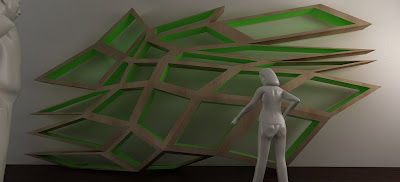## 25 January 2009

### VB script diagrid02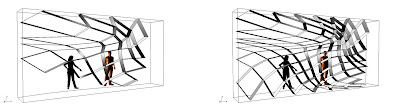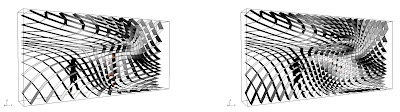## 24 January 2009

### VB script diagrid01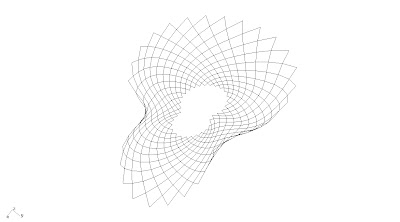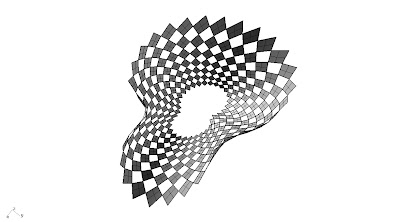Testing Visual Basic script in rhino grasshopper.

"Diagrid (a portmanteau of diagonal grid) is a design for constructing large buildings with steel that creates triangular structures with diagonal support beams. It requires less structural steel than a conventional steel frame. Hearst Tower reportedly uses 21 percent less steel than a standard design. The Diagrid also obviates the need for large corner columns and provides a better distribution of load in the case of a compromised building. Another building designed by Sir Norman Foster, 30 St Mary Axe, known as "the Gherkin", also makes use of this structural system."via Wikipedia

Class Grasshopper_Custom_Script
#Region "members"
Private app As MRhinoApp
Private doc As MRhinoDoc

Public A As System.Object
#End Region

Sub RunScript(ByVal pts As List(Of On3dPoint), ByVal u As Integer, ByVal v As Integer)

Dim arrPt(0 To u, 0 To v) As On3dPoint

Dim cnt As Integer = 0

For i As Int32 = 0 To u

For j As Int32 = 0 To v

arrPt(i, j) = pts(cnt)

cnt = cnt + 1

Next

Next

Dim lines As New List(Of OnCurve)()
'Point Culling
For i As Int32 = 0 To u - 2 Step 2

For j As Int32 = 0 To v - 2 Step 2
lines.Add(New OnLineCurve(arrPt(i + 1, j), arrPt(i + 2, j + 1)))
lines.Add(New OnLineCurve(arrPt(i + 2, j + 1), arrPt(i + 1, j + 2)))
lines.Add(New OnLineCurve(arrPt(i + 1, j + 2), arrPt(i, j + 1)))
lines.Add(New OnLineCurve(arrPt(i, j + 1), arrPt(i + 1, j)))

lines.Add(New OnLineCurve(arrPt(i + 2, j + 1), arrPt(i + 3, j + 2)))
lines.Add(New OnLineCurve(arrPt(i + 3, j + 2), arrPt(i + 2, j + 3)))
lines.Add(New OnLineCurve(arrPt(i + 2, j + 3), arrPt(i + 1, j + 2)))
lines.Add(New OnLineCurve(arrPt(i + 1, j + 2), arrPt(i + 2, j + 1)))

Next

Next

A = lines

End Sub

#Region "Additional methods and Type declarations"

#End Region
End Class